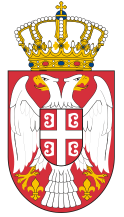Dokument se učitava

# Electrical EngineeringCourse code: 101007 | 7 ECTS credits

Basic information
Level of Studies: Undergraduate applied studies
Year of Study: 1
Semester: 1
Requirements:
Goal: Students are educated in basic knowledge in the field of Electrical Engineering.
Outcome: Students will be able to attend applied courses.
Contents of the course
Theoretical instruction:
1. 1. Electrostatics. Coulomb’s law. Quasi punctual charges. Electrostatic field vector.
2. 2. Electrostatics. Potential difference and voltage. Capacitors.
3. 3. DC current electrical circuits: Electrical current, Resistors, Ohm’s law, Jul’s law.
4. 4. DC current electrical circuits: Electrical power, Generators, Kirchhoff’s laws.
5. 5. DC current electrical circuits: Methods for resolving complex electrical circuits, Contour current method.
6. 6. DC current electrical circuits: Complex circuits transfigurations.
7. 7. DC current electrical circuits: Theorems for resolving complex electrical circuits, Tevenen’s theorem.
8. 8. DC current electrical circuits: Theorems for resolving complex electrical circuits, Superposition theorem.
9. 9. Magnetism: Magnetic field.
10. 10. Magnetism: Magnetic characteristics of materials, Magnetic induction.
11. 11. Magnetism: Inductive elements and Inductivity.
12. 12. AC current electrical circuits: AC current generators, Resistors in the AC current circuits.
13. 13. AC current electrical circuits: Inductive and Capacitive elements in the AC current circuits.
14. 14. AC current electrical circuits: Serial and parallel R, L, C connections.
15. 15. AC current electrical circuits: Methods and theorems for resolving complex electrical AC current circuits.
Practical instruction (Problem solving sessions/Lab work/Practical training):
1. Practical training program follows the lecture.
Textbooks and References
1. A. Đorđević: Osnovi elektrotehnike 1 – Elektrostatika, Akademska misao, Beograd, 2007.
2. A. Đorđević: Osnovi elektrotehnike 2 – Stalne struje, Akademska misao, Beograd, 2007
3. S. Krstić, I.Đukić:Zbirka zadataka iz elektrotehnike - Elektrostatika - Vremenski nepromenljive električne struje, VIŠER, Beograd, 2014.
4. S. Krstić, I.Đukić:Zbirka zadataka iz elektrotehnike - elektromagnetizam - vremenski promenljive električne struje, VIŠER, Beograd, 2010.
Number of active classes (weekly)
Lectures: 3
Practical classes: 3
Other types of classes: 0
Grading (maximum number of points: 100)
Pre-exam obligations
Points
activities during lectures
10
activities on practial excersises
0
seminary work
0
colloquium
50
Final exam
Points
Written exam
40
Oral exam
0© 2019 School of Electrical and Computer Engineering of Applied Studies, Belgrade
Vojvode Stepe 283, office@viser.edu.rs, +381 11 2471 099